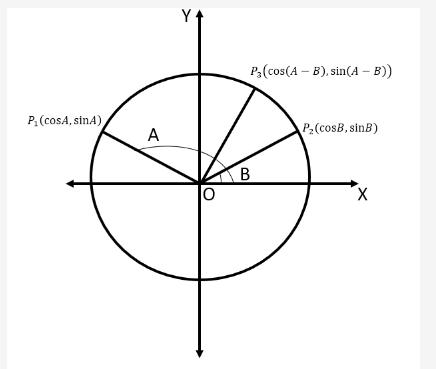# Prove that: (i) cos(A-B)= cosAcosB + sinAsinB (ii)cos(A+B)= cosAcosB - sinAsinB

Prove that:

(i) cos(A-B)= cosAcosB + sinAsinB
(ii)cos(A+B)= cosAcosB - sinAsinB